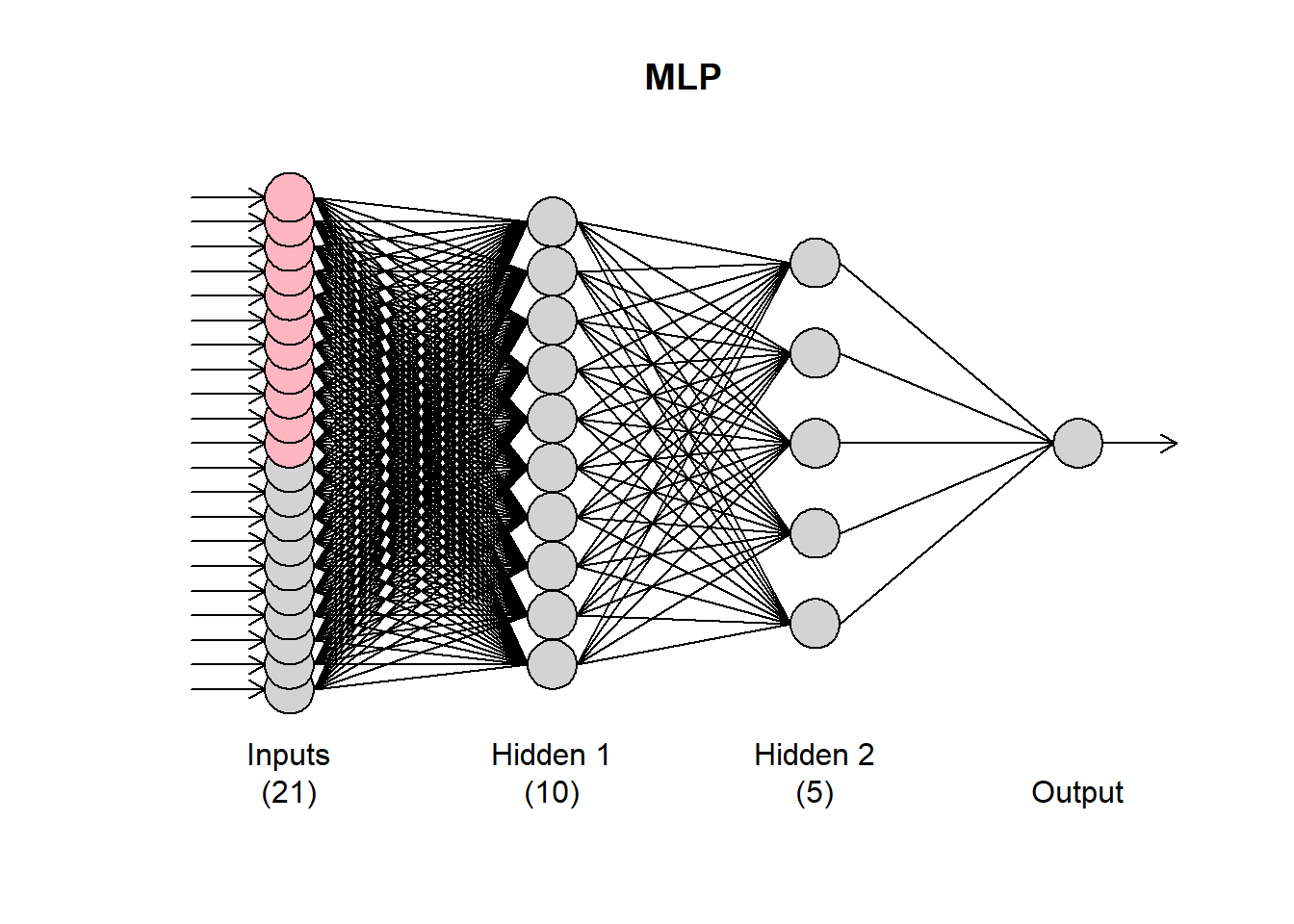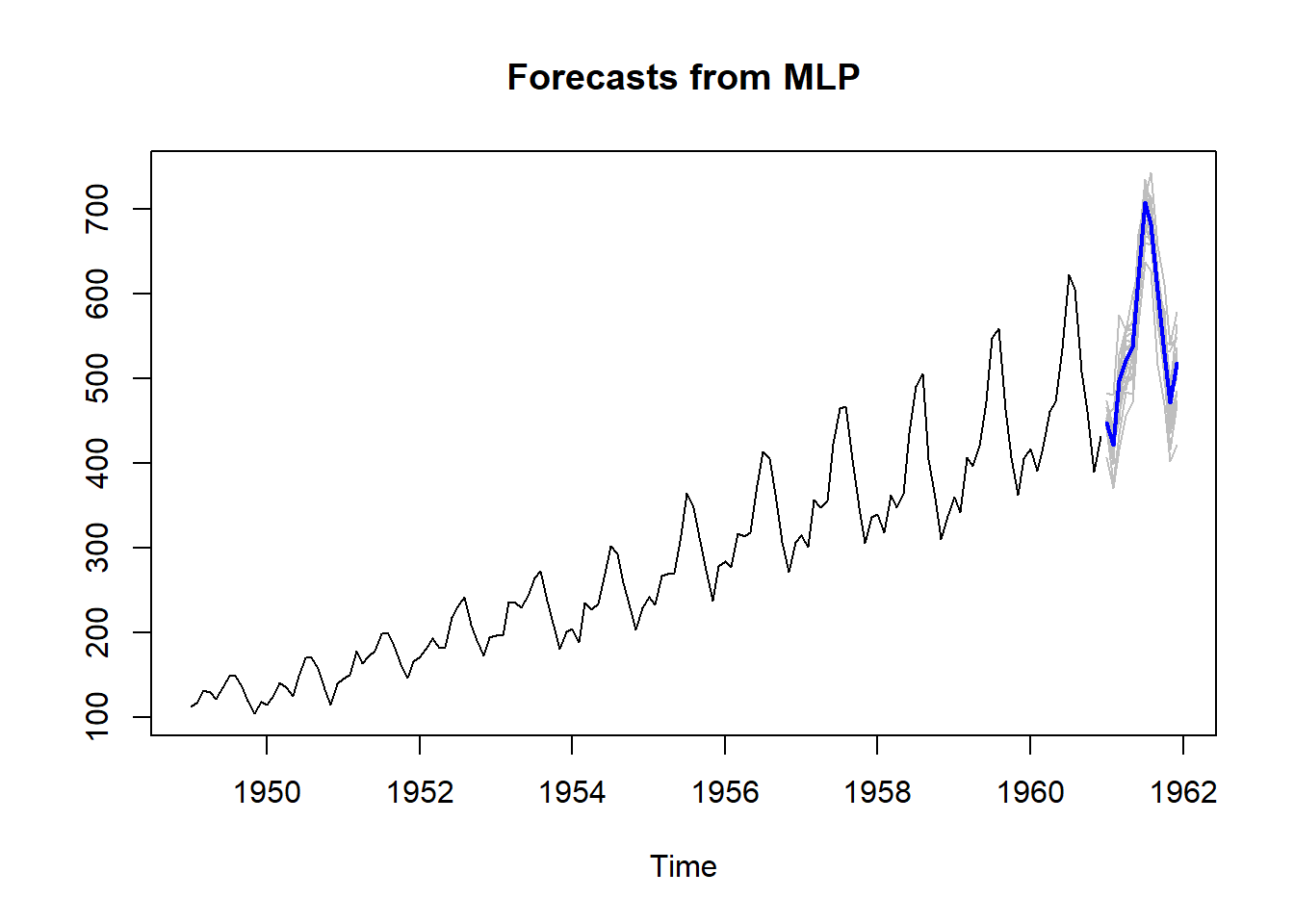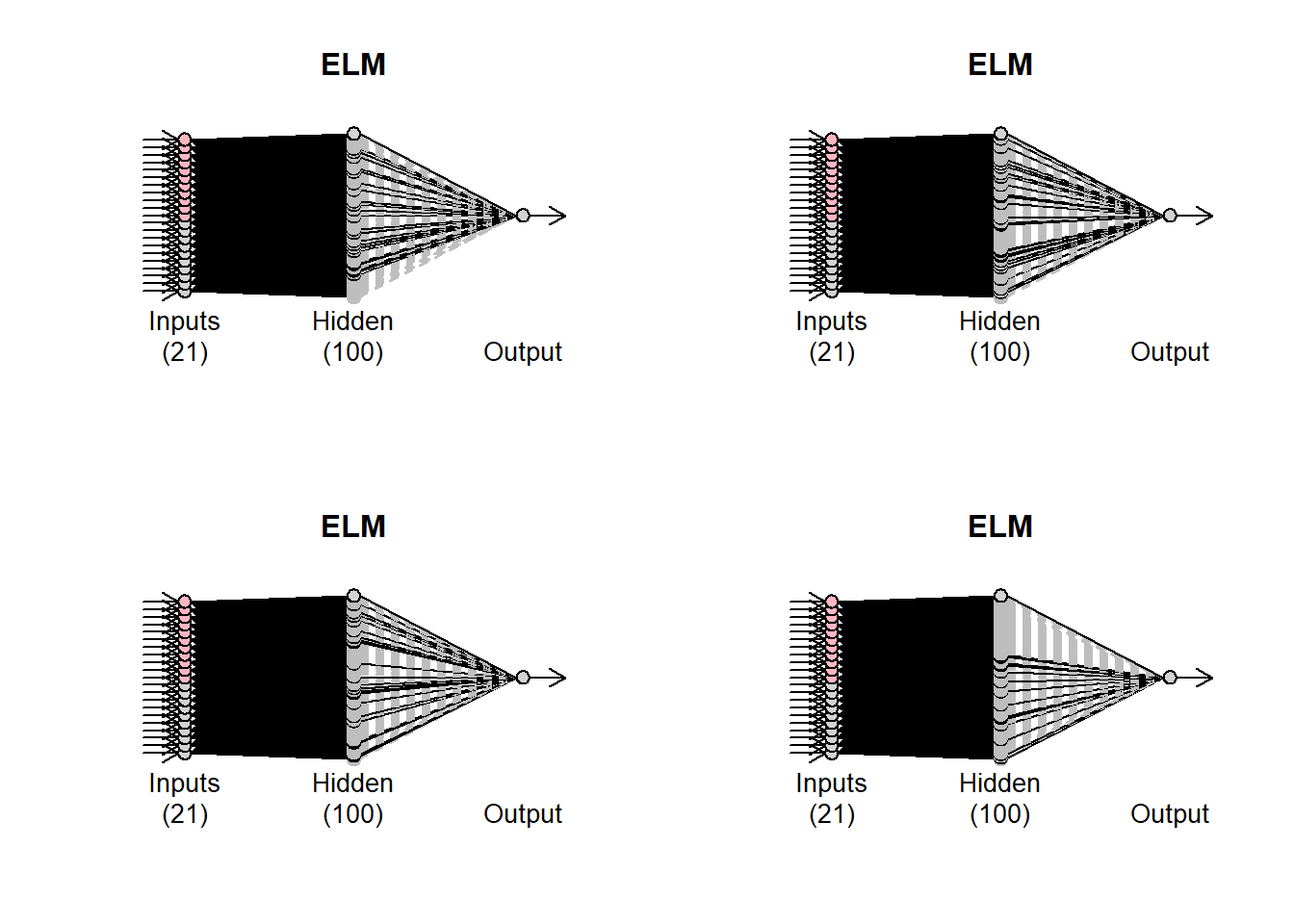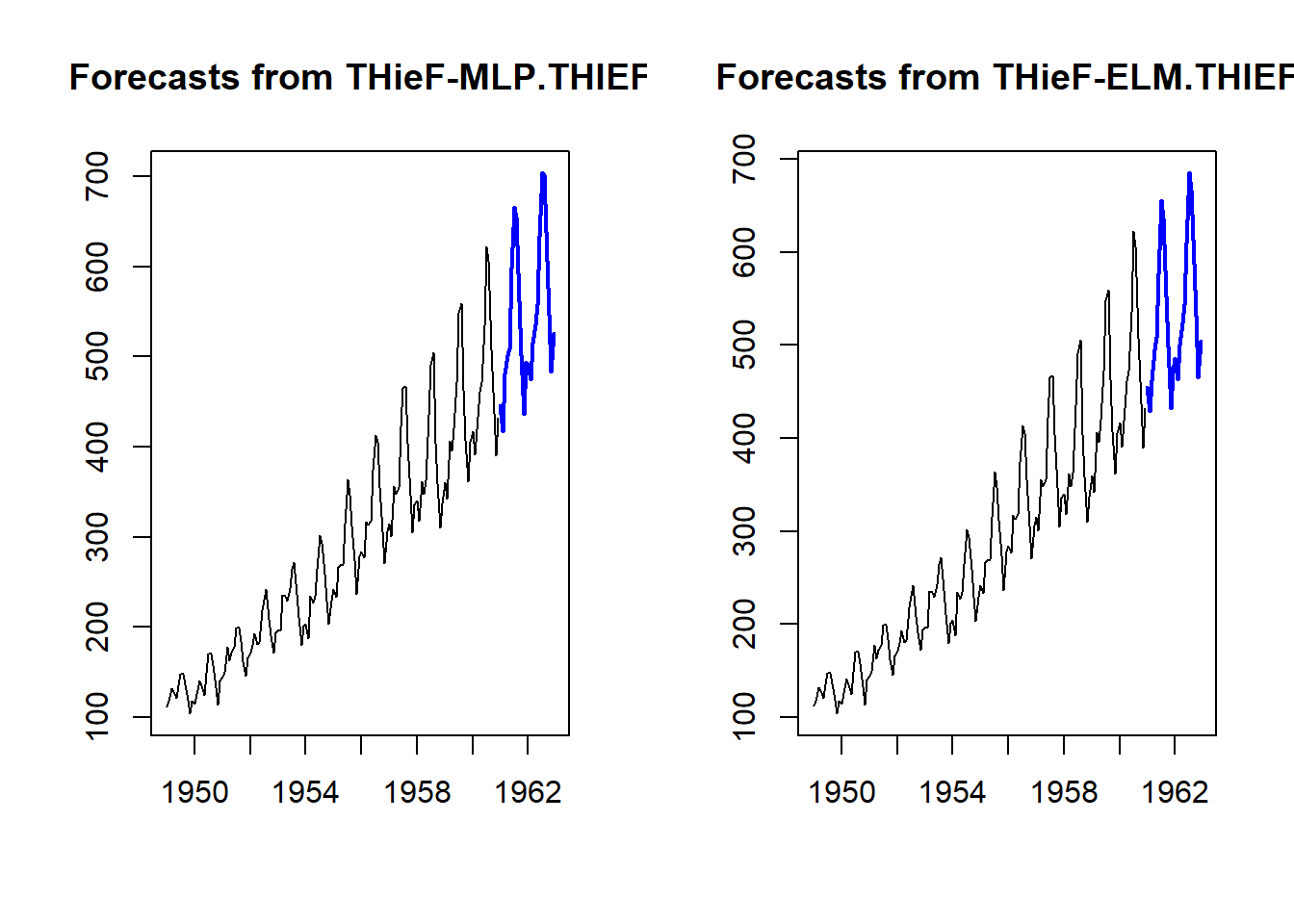# Tutorial for the nnfor R package

By | January 16, 2019
The nnfor (development version here) package for R facilitates time series forecasting with Multilayer Perceptrons (MLP) and Extreme Learning Machines (ELM). Currently (version 0.9.6) it does not support deep learning, though the plan is to extend this to this direction in the near future. Currently, it relies on the neuralnet package for R, which provides all the machinery to train MLPs. The training of ELMs is written within the nnfor package. Note that since neuralnet cannot tap on GPU processing, large networks tend to be very slow to train. nnfor differs from existing neural network implementations for R in that it provides code to automatically design networks with reasonable forecasting performance, but also provide in-depth control to the experienced user. The automatic specification is designed with parsimony in mind. This increases the robustness of the resulting networks, but also helps reduce the training time.

## Forecasting with MLPs

With the nnfor package you can either produce extrapolative (univariate) forecast, or include explanatory variables as well.

### Univariate forecasting

The main function is `mlp()`, and at its simplest form you only need to input a time series to be modelled.

``````library(nnfor)
fit1 <- mlp(AirPassengers)
print(fit1)``````
``````## MLP fit with 5 hidden nodes and 20 repetitions.
## Series modelled in differences: D1.
## Univariate lags: (1,2,3,4,5,6,7,8,10,12)
## Deterministic seasonal dummies included.
## Forecast combined using the median operator.
## MSE: 7.4939.``````

The output indicates that the resulting network has 5 hidden nodes, it was trained 20 times and the different forecasts were combined using the median operator. The `mlp()` function automatically generates ensembles of networks, the training of which starts with different random initial weights. Furthermore, it provides the inputs that were included in the network. This paper discusses the performance of different combination operators and finds that the median performs very well, the mode can achieve the best performance but needs somewhat larger ensembles and the arithmetic mean is probably best avoided! Another interesting finding in that paper is that bagging (i.e. training the network on bootstrapped series) or using multiple random training initialisations results in similar performance, and therefore it appears that for time series forecasting we can avoid the bootstrapping step, greatly simplifying the process. These findings are embedded in the default settings of nnfor.

You can get a visual summary by using the `plot()` function.

``plot(fit1)``The grey input nodes are autoregressions, while the magenta ones are deterministic inputs (seasonality in this case). If any other regressors were included, they would be shown in light blue.

The `mlp()` function accepts several arguments to fine-tune the resulting network. The `hd` argument defines a fixed number of hidden nodes. If it is a single number, then the neurons are arranged in a single hidden node. If it is a vector, then these are arranged in multiple layers.

``````fit2 <- mlp(AirPassengers, hd = c(10,5))
plot(fit2)``````We will see later on how to automatically select the number of nodes. In my experience (and evidence from the literature), conventional neural networks, forecasting single time series, do not benefit from multiple hidden layers. The forecasting problem is typically just not that complex!

The argument `reps` defines how many training repetitions are used. If you want to train a single network you can use `reps=1`, although there is overwhelming evidence that there is no benefit in doing so. The default `reps=20` is a compromise between training speed and performance, but the more repetitions you can afford the better. They help not only in the performance of the model, but also in the stability of the results, when the network is retrained. How the different training repetitions are combined is controlled by the argument `comb` that accepts the options `median`, `mean`, and `mode`. The mean and median are apparent. The mode is calculated using the maximum of a kernel density estimate of the forecasts for each time period. This is detailed in the aforementioned paper and exemplified here.

The argument `lags` allows you to select the autoregressive lags considered by the network. If this is not provided then the network uses lag 1 to lag `m`, the seasonal period of the series. These are suggested lags and they may not stay in the final networks. You can force that using the argument `keep`, or turn off the automatic input selection altogether using the argument `sel.lag=FALSE`. Observe the differences in the following calls of `mlp()`.

``mlp(AirPassengers, lags=1:24)``
``````## MLP fit with 5 hidden nodes and 20 repetitions.
## Series modelled in differences: D1.
## Univariate lags: (1,2,4,7,8,9,10,11,12,13,18,21,23,24)
## Deterministic seasonal dummies included.
## Forecast combined using the median operator.
## MSE: 5.6388.``````
``mlp(AirPassengers, lags=1:24, keep=c(rep(TRUE,12), rep(FALSE,12)))``
``````## MLP fit with 5 hidden nodes and 20 repetitions.
## Series modelled in differences: D1.
## Univariate lags: (1,2,3,4,5,6,7,8,9,10,11,12,13,18,21,23,24)
## Deterministic seasonal dummies included.
## Forecast combined using the median operator.
## MSE: 3.6993.``````
``mlp(AirPassengers, lags=1:24, sel.lag=FALSE)``
``````## MLP fit with 5 hidden nodes and 20 repetitions.
## Series modelled in differences: D1.
## Univariate lags: (1,2,3,4,5,6,7,8,9,10,11,12,13,14,15,16,17,18,19,20,21,22,23,24)
## Deterministic seasonal dummies included.
## Forecast combined using the median operator.
## MSE: 1.7347.``````

In the first case lags (1,2,4,7,8,9,10,11,12,13,18,21,23,24) are retained. In the second case all 1-12 are kept and the rest 13-24 are tested for inclusion. Note that the argument `keep` must be a logical with equal length to the input used in `lags`. In the last case, all lags are retained. The selection of the lags heavily relies on this and this papers, with evidence of its performance on high-frequency time series outlined here. An overview is provided in: Ord K., Fildes R., Kourentzes N. (2017) Principles of Business Forecasting 2e. Wessex Press Publishing Co., Chapter 10.

Note that if the selection algorithm decides that nothing should stay in the network, it will include lag 1 always and you will get a warning message: `No inputs left in the network after pre-selection, forcing AR(1)`.

Neural networks are not great in modelling trends. You can find the arguments for this here. Therefore it is useful to remove the trend from a time series prior to modelling it. This is handled by the argument `difforder`. If `difforder=0` no differencing is performed. For `diff=1`, level differences are performed. Similarly, if `difforder=12` then 12th order differences are performed. If the time series is seasonal with seasonal period 12, this would then be seasonal differences. You can do both with `difforder=c(1,12)` or any other set of difference orders. If `difforder=NULL` then the code decides automatically. If there is a trend, first differences are used. The series is also tested for seasonality. If there is, then the Canova-Hansen test is used to identify whether this is deterministic or stochastic. If it is the latter, then seasonal differences are added as well.

Deterministic seasonality is better modelled using seasonal dummy variables. by default the inclusion of dummies is tested. This can be controlled by using the logical argument `allow.det.season`. The deterministic seasonality can be either a set of binary dummies, or a pair of sine-cosine (argument `det.type`), as outlined here. If the seasonal period is more than 12, then the trigonometric representation is recommended for parsimony.

The logical argument `outplot` provides a plot of the fit of network.

The number of hidden nodes can be either preset, using the argument `hd` or automatically specified, as defined with the argument `hd.auto.type`. By default this is `hd.auto.type="set"` and uses the input provided in `hd` (default is 5). You can set this to `hd.auto.type="valid"` to test using a validation sample (20% of the time series), or `hd.auto.type="cv"` to use 5-fold cross-validation. The number of hidden nodes to evaluate is set by the argument `hd.max`.

``````fit3 <- mlp(AirPassengers, hd.auto.type="valid",hd.max=8)
print(fit3)``````
``````## MLP fit with 4 hidden nodes and 20 repetitions.
## Series modelled in differences: D1.
## Univariate lags: (1,2,3,4,5,6,7,8,10,12)
## Deterministic seasonal dummies included.
## Forecast combined using the median operator.
## MSE: 14.2508.``````

Given that training networks can be a time consuming business, you can reuse an already specified/trained network. In the following example, we reuse `fit1` to a new time series.

``````x <- ts(sin(1:120*2*pi/12),frequency=12)
mlp(x, model=fit1)``````
``````## MLP fit with 5 hidden nodes and 20 repetitions.
## Series modelled in differences: D1.
## Univariate lags: (1,2,3,4,5,6,7,8,10,12)
## Deterministic seasonal dummies included.
## Forecast combined using the median operator.
## MSE: 0.0688.``````

This retains both the specification and the training from `fit1`. If you want to use only the specification, but retrain the network, then use the argument `retrain=TRUE`.

``mlp(x, model=fit1, retrain=TRUE)``
``````## MLP fit with 5 hidden nodes and 20 repetitions.
## Series modelled in differences: D1.
## Univariate lags: (1,2,3,4,5,6,7,8,10,12)
## Deterministic seasonal dummies included.
## Forecast combined using the median operator.
## MSE: 0.``````

Observe the difference in the in-sample MSE between the two settings.

Finally, you can pass arguments directly to the `neuralnet()` function that is used to train the networks by using the ellipsis `...`.

To produce forecasts, we use the function `forecast()`, which requires a trained network object and the forecast horizon `h`.

``````frc <- forecast(fit1,h=12)
print(frc)``````
``````##              Jan         Feb         Mar         Apr         May
## 1961 447.3392668 421.2532515 497.5052166 521.1640683 537.4031708
##              Jun         Jul         Aug         Sep         Oct
## 1961 619.5015908 707.8790407 681.0523280 602.8467629 529.0477736
##              Nov         Dec
## 1961 470.8292734 517.6762262``````
``plot(frc)``The plot of the forecasts provides in grey the forecasts of all the ensemble members. The output of `forecast()` is of class `forecast` and those familiar with the forecast package will find familiar elements there. To access the point forecasts use `frc\$mean`. The `frc\$all.mean` contains the forecasts of the individual ensemble members.

### Using regressors

There are three arguments in the `mlp()` function that enable to use explanatory variables: `xreg`, `xreg.lags` and `xreg.keep`. The first is used to input additional regressors. These must be organised as an array and be at least as long as the in-sample time series, although it can be longer. I find it helpful to always provide `length(y)+h`. Let us suppose that we want to use a deterministic trend to forecast the time series. First, we construct the input and then model the series.

``````z <- 1:(length(AirPassengers)+24) # I add 24 extra observations for the forecasts
z <- cbind(z) # Convert it into a column-array
fit4 <- mlp(AirPassengers,xreg=z,xreg.lags=list(0),xreg.keep=list(TRUE),
# Add a lag0 regressor and force it to stay in the model
difforder=0) # Do not let mlp() to remove the stochastic trend
print(fit4)``````
``````## MLP fit with 5 hidden nodes and 20 repetitions.
## Univariate lags: (1,4,5,8,9,10,11,12)
## 1 regressor included.
## - Regressor 1 lags: (0)
## Deterministic seasonal dummies included.
## Forecast combined using the median operator.
## MSE: 32.4993.``````

The output reflects the inclusion of the regressor. This is reflected in the plot of the network with a light blue input.

``plot(fit4)``Observe that `z` is organised as an array. If this is a vector you will get an error. To include more lags, we expand the `xreg.lags`:

``mlp(AirPassengers,difforder=0,xreg=z,xreg.lags=list(1:12))``
``````## MLP fit with 5 hidden nodes and 20 repetitions.
## Univariate lags: (1,4,5,8,9,10,11,12)
## Deterministic seasonal dummies included.
## Forecast combined using the median operator.
## MSE: 48.8853.``````

Observe that nothing was included in the network. We use the `xreg.keep` to force these in.

``mlp(AirPassengers,difforder=0,xreg=z,xreg.lags=list(1:12),xreg.keep=list(c(rep(TRUE,3),rep(FALSE,9))))``
``````## MLP fit with 5 hidden nodes and 20 repetitions.
## Univariate lags: (1,4,5,8,9,10,11,12)
## 1 regressor included.
## - Regressor 1 lags: (1,2,3)
## Deterministic seasonal dummies included.
## Forecast combined using the median operator.
## MSE: 32.8439.``````

Clearly, the network does not like the deterministic trend! It only retains it, if we force it. Observe that both `xreg.lags` and `xreg.keep` are lists. Where each list element corresponds to a column in `xreg`. As an example, we will encode extreme residuals of `fit1` as a single input (see this paper for a discussion on how networks can code multiple binary dummies in a single one). For this I will use the function `residout()` from the tsutils package.

``````if (!require("tsutils")){install.packages("tsutils")}
library(tsutils)
loc <- residout(AirPassengers - fit1\$fitted, outplot=FALSE)\$location
zz <- cbind(z, 0)
zz[loc,2] <- 1
fit5 <- mlp(AirPassengers,xreg=zz, xreg.lags=list(c(0:6),0),xreg.keep=list(rep(FALSE,7),TRUE))
print(fit5)``````
``````## MLP fit with 5 hidden nodes and 20 repetitions.
## Series modelled in differences: D1.
## Univariate lags: (1,2,3,4,5,6,7,8,10,12)
## 1 regressor included.
## - Regressor 1 lags: (0)
## Deterministic seasonal dummies included.
## Forecast combined using the median operator.
## MSE: 7.2178.``````

Obviously, you can include as many regressors as you want.

To produce forecasts, we use the `forecast()` function, but now use the `xreg` input. The way to make this work is to input the regressors starting from the same observation that was used during the training of the network, expanded as need to cover the forecast horizon. You do not need to eliminate unused regressors. The network will take care of this.

``frc.reg <- forecast(fit5,xreg=zz)``

## Forecasting with ELMs

To use Extreme Learning Machines (EMLs) you can use the function `elm()`. Many of the inputs are identical to `mlp()`. By default ELMs start with a very large hidden layer (100 nodes) that is pruned as needed.

``````fit6 <- elm(AirPassengers)
print(fit6)``````
``````## ELM fit with 100 hidden nodes and 20 repetitions.
## Series modelled in differences: D1.
## Univariate lags: (1,2,3,4,5,6,7,8,10,12)
## Deterministic seasonal dummies included.
## Forecast combined using the median operator.
## Output weight estimation using: lasso.
## MSE: 91.288.``````
``plot(fit6)``Observe that the plot of the network has some black and some grey lines. The latter are pruned. There are 20 networks fitted (controlled by the argument `reps`). Each network may have different final connections. You can inspect these by using `plot(fit6,1)`, where the second argument defines which network to plot.

``````par(mfrow=c(2,2))
for (i in 1:4){plot(fit6,i)}
par(mfrow=c(1,1))``````

How the pruning is done is controlled by the argument.`type` The default option is to use LASSO regression (type=“lasso”). Alternatively, use can use “ridge” for ridge regression, “step” for stepwise OLS and “lm” to get the OLS solution with no pruning.

The other difference from the `mlp()` function is the `barebone` argument. When this is `FALSE`, then the ELMs are built based on the neuralnet package. If this is set to `TRUE` then a different internal implementation is used, which is helpful to speed up calculations when the number of inputs is substantial.

To forecast, use the `forecast()` function in the same way as before.

``forecast(fit6,h=12)``
``````##              Jan         Feb         Mar         Apr         May
## 1961 449.0982276 423.0329121 455.7643540 488.5096713 499.3616506
##              Jun         Jul         Aug         Sep         Oct
## 1961 567.7486203 645.6309202 622.8311280 543.9549163 495.8305278
##              Nov         Dec
## 1961 429.9258197 468.7921570``````

## Temporal hierarchies and nnfor

People who have followed my research will be familiar with Temporal Hierarchies that are implemented in the package thief. You can use both `mlp()` and `elm()` with `thief()` by using the functions `mlp.thief()` and `elm.thief()`.

``````if (!require("thief")){install.packages("thief")}
library(thief)
thiefMLP <- thief(AirPassengers,forecastfunction=mlp.thief)
# Similarly elm.thief:
thiefELM <- thief(AirPassengers,forecastfunction=elm.thief)
par(mfrow=c(1,2))
plot(thiefMLP)
plot(thiefELM)
par(mfrow=c(1,1))``````This should get you going with time series forecasting with neural networks.

Happy forecasting!

## 26 thoughts on “Tutorial for the nnfor R package”

1.Jesseca

Good Day, great work. I’ve been following you with your other articles.Thank you for this, I have now the lead on how to perform NARX in R. But I am not really confident on my forecast

Can you help me?

2.Jesseca

I am an undergraduate of Bachelor in Statistics and my undergrad thesis is all about the comparison of the ARIMAX model and NARX model(forecasting). Now I am working for the NARX model. And I have a hard time understanding it. But thanks to your article I can now perform it in R. The only problem is that, in Y axis from “Forecasts in MLP”, it shows (-2.0, -1.0, 0.0, 1.0) instead of my Yield Variables, why did it changed? I followed every codes.

1.Nikos Post author

Hi Jesseca, hmm that is strange. Does the rest of the plot look okay? It seems to be that something has gone off with the scaling of the variables. What is the range of your target variable?

3.Cristian Valdez

Thank you for this amazing post.

4.Atif

in highlighted foretasted area , there are many grey lines , what exactly the meaning of that lines.

1.Nikos Post author

The grey lines are the forecasts of the individual ensemble members. The blue line is the ensemble forecast.

5.Jc

Hi there, I’ve been following your posts and your articles about the neural network, uhmm can I ask if do you have any recommendation, a book or an article somehow, that compares the result if I run the neural network in R software and in MATLAB? I just want to find if it gives the same result if I use the same data. Thank you

1.Nikos Post author

You will find that the results are substantially different. This is mainly to different implentation and options in training the networks. I am not aware of any article or blog post that directly compares these.

6.Alphonse

Hi everyone. I have a question.
Multi-step forecasts in nonlinear model is not tractable. Therefore, forecasts can be obtained using 3 different approaches: the naïve approach, bootstrap and Monte Carlo. Which one is used when we have

forecast(fit,h=10)

and how to obtain the forecasts in the two other approaches?

1.Nikos Post author

I am not entirely sure what you are referring to, but at least in nnfor there is no bootstrap or monte carlo option. I cannot see any reason that you could not write a wrapper function around existing mlp functionality to do that.

7.Jesseca

Hi there, been following your blog quite a while now. do you have any recommendation a book or an article somehow that compares NARX neural network in matlab and in R? Hoping for your reply. Thank you

1.Nikos Post author

Unfortunately not, but from experience I can say that the implementation of neural networks in matlab is quite good, in particular the details on the training algorithms. As for the NARX, I had found the matlab functions somewhat restrictive, but this was years ago! Good chance now this is no longer the case. R is lacking a flexible package for neural networks, especially when it comes to training. Deep learning libraries, are quite good for deep learning, but do not have all the tools that would be handy for conventional MLPs – not that they should!

8.Gabriel Lins

Dear Nikos, It’s possible to implement cross validation using exogenous variables, through the “forecast” package? (tsCV function)
I tried several scripts, but completely fail.

1.Nikos Post author

I have not tried this, but I cannot see why it would not be possible to write a wrapper function to do this. I am not using tsCV myself, so I am not sure of the internal workings of the function, but in principle there should be nothing to stop you from doing it. However, I would expect tremendous search times for cross-validating inputs for neural networks!

9.Alina

Do you know if and even why the number of forecasts depends on the frequency of the trained time series? For example you used monthly data and it produced 24 forecasts.. i tried to di the seme with daily time series and it produced just 1 forecast.. when i would like the model to forecast 120 days

1.Nikos Post author

There is a setting for the horizon (h), so you can choose what you need!

10.Sabyr

Nikos, thank you for the amazing tutorial.
I have couple questions: what transfer function is used in mlp sigmoid or hyperbolic?

Likewise, when trying to use elm on daily data (daily vol proxies), elm uses only one hidden node and the fit is just a straight line. input for elm() is a ts with frequency 1 and length 755 (~3 years).

1.Nikos Post author

By default it is using hyperbolic tangent. As for the ELM question, this could be due to many reasons. It could be due to the information contained in the time series, but most probably the automatic routines don’t do a great job on your time series. If it prefers a single hidden node, it would appear to me that it cannot pick up a lot of information from the time series. I would start by manually setting different input lags.

11.Casper

Do you have any literature or blogs that provide guidance on how to select the lags?
As you showed in the example above, choosing your own lags can achieve better performance than the default option.

1.Nikos Post author

Have a look at the references provided in the nnfor package. I have a couple of “ancient” papers on this that seems to do the trick for MLPs.

12.Guilhermino Neto

Dear Professor Kourentzes,

First of all, congratulations for the development of the package. I see R really lacks on Neural Networks compared to other languages and platforms, and so your work is of great value for us, R users.

I am a doctorate student on Computer Modelling, and my research is focused on time series forecasting (currently working on electrical load forecasting) and applied statistics. I’ve just started trying nnfor, but I could find no information on number of epochs, the stopping criteria and the optimization algorithm. Is that possible to make these settings? If not, how can I know what the function is doing?

Also, how can I build a mlp with several outputs? Would I have to use a mts object as my x parameter on the mlp() function?

Thank you for your attention. My best regards.

1.Nikos Post author

Thank you! Unfortunately the current implementation does not offer a multiple output option. At the core of nnfor I am using the neuralnet package for R, which details all the training options. You can pass those to mlp() as well. As for the current training settings, the defaults from neuralnet are used, as to my experiments they work fine!

13.Alphonse Malonda Tsumbu

The multiple step forecast in nonlinear model is intractable because we loose normality after the first step. However it can still be acheived frombootstrap, Monte Carlo or Naive method. I want to know which of those methods is used by typing the comment forecast (mlp_object). I suspect those are Naive forecast but I prefer to be sure. Thank you!

1.Nikos Post author

Yes and no! 🙂 Depends how you generate the multi-step ahead forecast. I am not sure why you need to assume normality here, nor why bootstrapping would be an effective way to deal with forecasts of nonlinear models. Keep in mind that you have not observed the complete nonlinearity captured by the network anyway and you hope to remain in regions of values that you have more or less seen in the past. If that is the case then bootstrapping makes some sense, as many other approaches, but none is “correct”!

14.Yunus Kara

Dear Professor Nikolaos Kourentzes
I want to MLP result only positive (not negative). But ı don’t do this. How are do this?

1.Nikos Post author

I am not aware of a standard algorithm that does that, so what I usually do is to is replace negative values with zeros after the forecast is generated. If your actual values are very close to zero then this may make some sense. If your actuals are substantially different from zero, then getting negative forecasts might be due to model misspecification.